Courses

# Inequalities JEE Notes | EduRev

## Mathematics (Maths) Class 11

Created by: Tarun Kaushik

## JEE : Inequalities JEE Notes | EduRev

The document Inequalities JEE Notes | EduRev is a part of the JEE Course Mathematics (Maths) Class 11.
All you need of JEE at this link: JEE

Inequalities

Let a and b be real numbers. If a – b is negative, we say that a is less than b (a < b) and if a – b is positive, then a is greater than b (a > b).

Important Points to be Remembered

(i) If a > b and b > c, then a > c. Generally, if a1 > a2, a2 > a3,…., an – 1 > an, then a1 > an.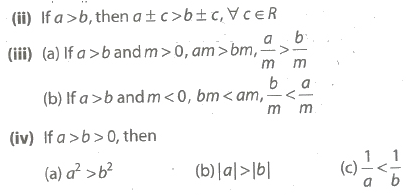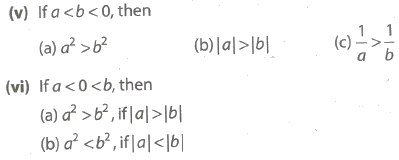(vii) If a < x < b and a, b are positive real numbers then a2 < x2 < b2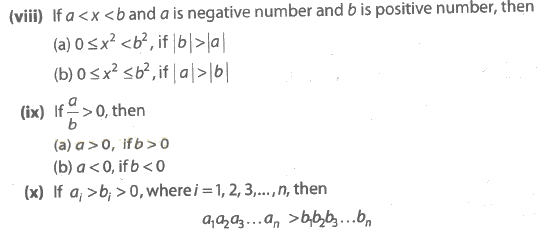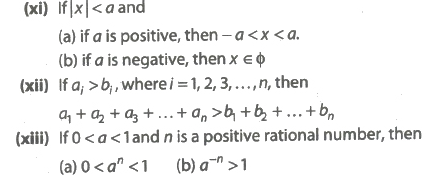Important Inequality

1. Arithmetico-Geometric and Harmonic Mean Inequality

(i) If a, b > 0 and a ≠ b, then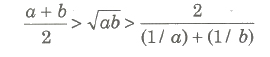(ii) if ai > 0, where i = 1,2,3,…,n, then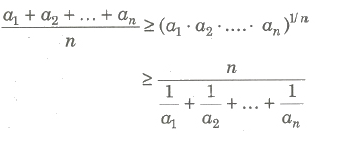(iii) If a1, a2,…, an are n positive real numbers and m1, m2,…,mn are n positive rational numbers, then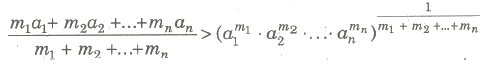i.e., Weighted AM > Weighted GM

(iv) If a1, a2,…, an are n positive distinct real numbers, then

(a)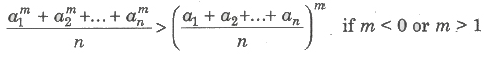(b)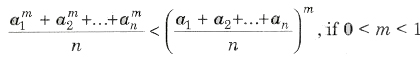(c) If a1, a2,…, an and b1, b2,…, bn are rational numbers and M is a rational number, then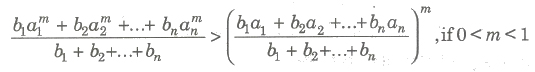(d)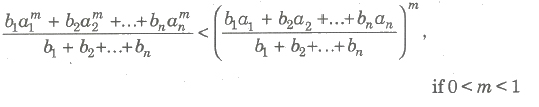(v) If a1, a2, a3,…, an are distinct positive real numbers and p, ,q, r are natural numbers, then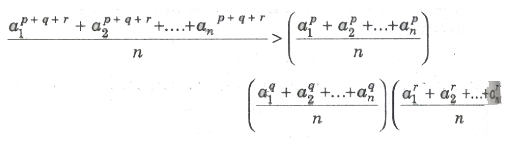2. Cauchy – Schwartz’s inequality

If a1, a2,…, an and b1, b2,…, bn are real numbers, such that

(a1b1 + a2b2 + …+ anbn)2 ≤ (a12 + a22 + …, an2) * (b12 + b22 + …, bn2)

Equality holds, iff a1 / b1 = a2 / b2 = an / bn

3. Tchebychef’s Inequality

Let a1, a2,…, an and b1, b2,…, bn are real numbers, such that

(i) If a1 ≤ a2 ≤ a3 ≤… ≤ an and b1 ≤ b2 ≤ b3 ≤… ≤ bn, then

n(a1b1 + a2b2 + a3b3 + …+ anbn) ≥ (a1 + a2 + …+ an) (b1 + b2 + …+ bn)

(ii) If If a1 ≥ a2 ≥ a3 ≥… ≥ an and b1 ≥ b2 ≥ b3 ≥… ≥ bn, then

n(a1b1 + a2b2 + a3b3 + …+ anbn) ≤ (a1 + a2 + …+ an) (b1 + b2 + …+ bn)

4. Weierstrass Inequality

(i) If a1, a2,…, an are real positive numbers, then for n ≥ 2

(1 + a1)(1 + a2) … (1 + an) > 1 + a1 + a2 + … + an

(ii) If a1, a2,…, an are real positive numbers, then

(1 – a1)(1 – a2) … (1 – an) > 1 – a1 – a2 – … – an

5. Logarithm Inequality

(i) (a) When y > 1 and logy x > z ⇒ x > yz

(b) When y > 1 and logy x < z ⇒ 0 < x < yz

(ii) (a) When 0 < y < 1 and logy x > z ⇒ 0 < x < yz

(b) hen 0 < y < 1 and logy x < z ⇒ x > yz

Application of Inequalities to Find the Greatest and Least Values

(i) If xl,x2,…,xn are n positive variables such that xl + x2 +…+ xn = c (constant), then the product xl * x2 *….* xn is greatest when x1 = x2 =… =xn = c/n and the greatest value is (c/n)n.

(ii) If xl,x2,…,xn are positive variables such that xl,x2,…,xn = c (constant), then the sum xl + x2 +….+ xn is least when x1 = x2 =… =xn = c1/n and the least value of the sum is n (c1/n).

(iii) If xl,x2,…,xn are variables and ml,m2,…,mn are positive real number such that xl + x2 +….+ xn = c (constant), then xlml * x2m2 *… * xnmn is greatest, when

xl / ml = x2 / m2 =…= xn / mn

= xl + x2 +….+ xn / ml + m2 +….+ mn

,

,

,

,

,

,

,

,

,

,

,

,

,

,

,

,

,

,

,

,

,

;